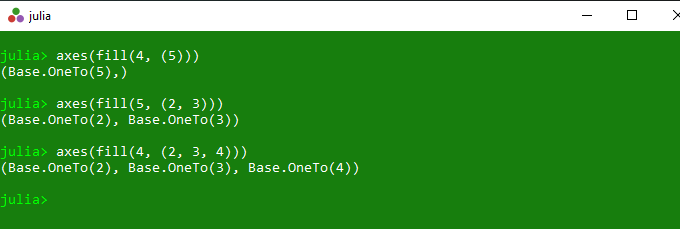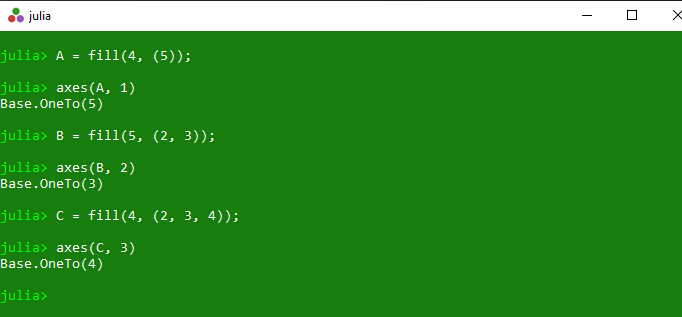Related Articles

# Getting tuple or range of the valid indices in Julia – axes() Method

• Last Updated : 26 Mar, 2020

The `axes()` is an inbuilt function in julia which is used to return the tuple or valid range of valid indices for specified array.

Syntax:
axes(A)
or
axes(A, d)

Parameters:

• A: Specified array.
• d: Specified dimension.

Returns: It returns the tuple or valid range of valid indices for specified array.

Example 1:

 `# Julia program to illustrate ``# the use of Array axes() method`` ` `# Getting tuple for 1D array of size 5``# with each element filled with value 4``println(axes(fill(``4``, (``5``))))`` ` `# Getting tuple for 2D array of size 2X3  ``# with each element filled with value 5  ``println(axes(fill(``5``, (``2``, ``3``))))`` ` `# Getting tuple for 3D array of size 2X3X4``# with each element filled with value 4``println(axes(fill(``4``, (``2``, ``3``, ``4``))))`

Output:Example 2:

 `# Julia program to illustrate ``# the use of Array axes() method`` ` `# Getting tuple for 1D array of size 5``# with each element filled with value 4``# along with dimension of 1``A ``=` `fill(``4``, (``5``));``println(axes(A, ``1``))`` ` `# Getting tuple for 2D array of size 2X3  ``# with each element filled with value 5  ``# along with dimension of 2``B ``=` `fill(``5``, (``2``, ``3``));``println(axes(B, ``2``))`` ` `# Getting tuple for 3D array of size 2X3X4``# with each element filled with value 4``# along with dimension of 3``C ``=` `fill(``4``, (``2``, ``3``, ``4``));``println(axes(C, ``3``))`

Output:My Personal Notes arrow_drop_up